Random Effects - - Fixed Effects Example:

Summary

 Least Squares Dummy Variables Models Model Specification RSS Log Likelihood 1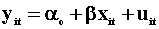5465 -188.3 2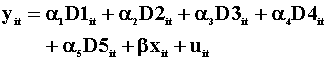4827 -185.2 3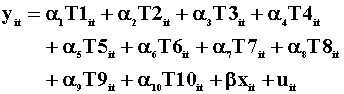3380 -176.3 4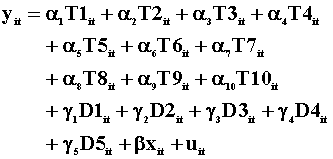2663 -170.3F-test Between LSDV Models Degrees of Freedom in Parentheses( ) 95% Critical Value in Italics 2 3 4 1 1.45 (4,44) 2.58 2.67 (9,39) 2.13 2.83 (13,35) 2.04 2 3.16 (9,35) 2.16 3 2.35 (4,35) 2.64
On the basis of the tabulated results:
We prefer model 1 to model 2: The simple model is better.
We prefer model 3 to model 1: The null that the coefficients on the time dummies are all zero is rejected.
We prefer model 4 to model 1: The full set of dummy coefficients are not all zero.
We prefer model 4 to model 2: The model with all of the dummies is preferred to the model with just individual effects.
We prefer model 3 to model 4: The model with just time dummies is preferred to the model with both time and person dummies.

The most preferred model is 3, with just time effects.Random Effects Models: Chi-square Tests Degrees of Freedom and 95% Critical Values are in Parentheses Model Specification Estimates of Variance Components LM for Model j versus Model 1 Hausman for Model j versus Model j-3 5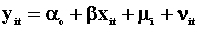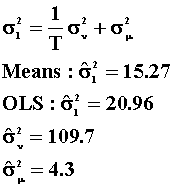.063 (1, 3.84) 1.19 (1) 6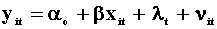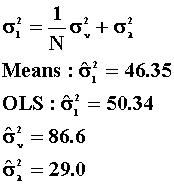4.43 (1) 1.24 (1) 7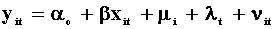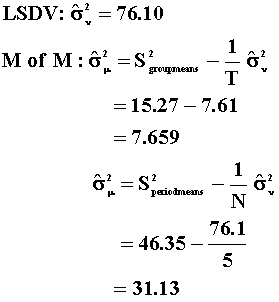4.49 (2,5.99) 2.91 (1)On the basis of the LM Test:

We prefer model 1 to model 5: The random individual effect is zero.
We prefer model 6 to model 1: The random time effect is not zero.
We prefer model 1 to model 7: We cannot reject the null hypothesis that both the random individual and time effects are zero.

The only REM preferred to OLS is model 6. OLS is better than either of the remaining two specifications.

On the basis of the Hausman Test:

We prefer model 5 to model 2: The random individual effect model is better than the individual fixed effect model.
We prefer model 6 to model 3: The random time effects model is better than fixed time effects model.
We prefer model 7 to model 4: The random effects model is better.

When given a choice, we never pick the fixed effects model.CONCLUSION

From the three groups of tests we have the following intransitivity involving model 7: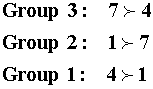so we'll eliminate 7 from the competition. From the different sets of tests we can establish the following ordering;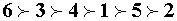So we'll choose model 6 as the best model.

Home